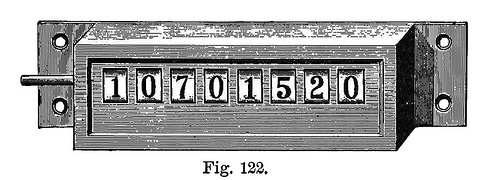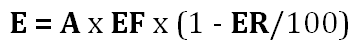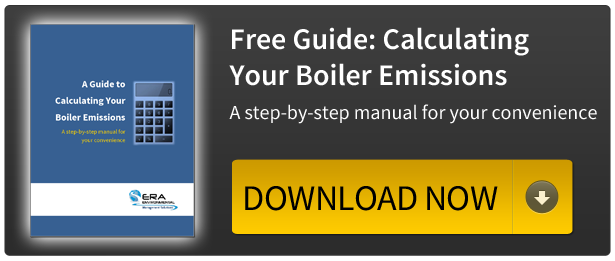<img src="//bat.bing.com/action/0?ti=5321010&amp;Ver=2" height="0" width="0" style="display:none; visibility: hidden;">

# How To Calculate Your Boiler MACT Emissions: A Free GuideLately, we have been getting a lot of comments and questions regarding the EPA's new Boiler Boiler MACT rules. This is mainly due to the confusion surrounding the regulations and how they'll apply to different facilities. One of the most popular questions we get is:

"How do I calculate my boiler emissions?"

Calculating your emissions is no easy task. When it comes time to report, calculating emissions is one of the biggest time drains there is. Not because the formulas you will need to use are overly complex, but simply because there is so much data to collect, manipulate, convert, record, and report.

Even if you have just one boiler, you may need to calculate emissions for a substantial number of hazardous air pollutants. The time commitment it could take to calculate the air emissions from many different types of boilers located across several facilities can be intimidating.

In order to calculate the emissions from a single boiler source the EPA uses the following equation:You'll need to provide the following information from your facility records:

• A the total amount of fuel used
• EF the emission factor for the specific HAP you are calculating for your particular boiler for that particular fuel
• ER the emission reduction value of your existing control technology

There are many variations of this formula depending on the type of boiler you use, the fuel you burn, if ash or sulphur is involved, and the emission factor data you are using.

To make it easier for you, ERA has prepared a free step-by-step guide detailing how you can calculate all of your boiler emissions by yourself. Our guide contains a complete list of the formula variations, links to a free online database of the most common emission factors, and tips on how to remove the hassle from doing calculations.
Download the guide today to recieve the information you'll need to:

• Understand the formulas you will need to use
• Know which formula to use for each type of boiler or emission factor
• Find the data you'll use in your calculations
• Remove the hassle from calculating your boiler emissions